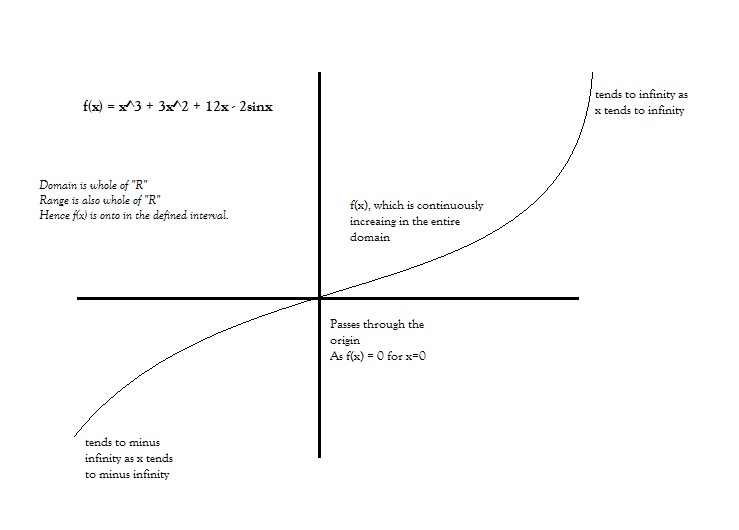# If f(x)=x3+3x2+12x-2sinx,where f is a function from R to R,then1.f(x) is many-one and onto2.f(x) is one-one and onto3.f(x) is one-one and into4.f(x) is many-one and into12 years ago

f(x) is many one and onto

11 years ago

Hi Meanks,

Solving this question, we would take the Differential Calculus approach.

Let y = f(x)

Then we have dy/dx = 3x^2 + 6x + 12 - 2cosx

Now, take a look at 3x^2 + 6x + 12, the minimum value of which is 9, when x = -1. And hence dy/dx is always positive (since cosx can take max value of 1, so dy/dx should always be positive)

Which will tell us that y = f(x) is an increasing function in its entire Domain R.

Which would give us that f is one-one function (as continuously increasing functions are always one-one because a line drawn parallel to the y-axis can intersect the graph in only one point)

Next also clearly f(x) is continuos, and also f(x) would tend to infinity when x tends to infinity, and f(x) would tend to -infinity when x tends to -infinity.

So the graph would be something like this:And so f(x) is both one-one and onto. Which is option (2).

Hope that hepls,

All the best,

Regards,